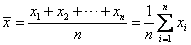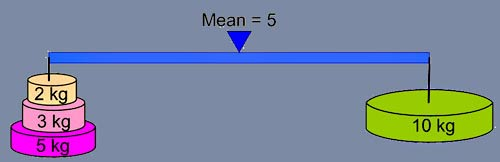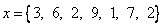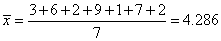## Arithmetic mean

Arithmetic mean is total value of data divided by the number of sample.

Other name: Average , Mean , Arithmetic average

Formula:Property of Arithmetic mean:

• A half of the input values are higher than arithmetic mean, and half of the other input values are lower than this mean. Arithmetic mean serves as the balancing point of input values. Note that it is the values that are divided into two, not the number of input values, as shown in the following figure.Example:The following interactive program computes Arithmetic mean of a list of numbers separated by comma. Feel free to change the input values. What happen when you have an outlier (say, a very big number or very small number compared to the rest of the data)? Do you think Arithmetic mean is robust against outlier or susceptible to error?

Input list of numbers separated by comma, then press the button "Get Arithmetic Mean". The program will compute directly when you change the input data.

Preferable reference for this tutorial is

Teknomo, Kardi (2015) Mean and Average. https:\\people.revoledu.com\kardi\tutorial\BasicMath\Average\